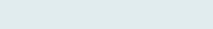# Sumproduct with Condition in Excel

Adding multiple rows if criteria met and then on certain conditions SUMPRODUCT functions are being used in Excel. In case you want to sum of the product based on condition, you can apply this formula.

QUERY

We have columns Price and Invoice quantity. Our assignment is to add all of the same quantity and then multiply them with another column, i.e. price in the given range and returns the addition of the products.

Formula to implement:

=SUMPRODUCT(SUMIF(\$E\$3:\$E\$14,\$B\$3:\$B\$8,\$F\$3:\$F\$14),\$C\$3:\$C\$8)

Functions applicable:

We will be creating SUMIF formula along with SUMPRODUCT formula in Excel:

• SUMPRODUCT: Is a function in excel that multiplies in a range of cells or arrays and returns the sum of products. It first multiplies and then adds values of the input arrays.
• SUMIF:  This function adds all numbers in a range of cells that meet specific criteria.

Formula Explanation (Step by Step)

You can also debug formula using shortcut F9 function key or under formulas tab click on evaluate the formula. Excel start calculating from the innermost parenthesis and then work outwardLet’s break the formula into multiple small steps to understand its application.

First formula:

Step 1: SUMIF(\$E\$3:\$E\$14,\$B\$3:\$B\$8,\$F\$3:\$F\$14)

Result:(17+15=32, 13+12=25, 32+10=42, 18+16=34, 20+25=45, 23+14=37)

It returns sum in the range (F3 to F14) based on the criteria (B3 to B8) i.e. with a similar text or a name for example all invoice quantity of table retruns (32), chair (25) and so on.

Second formula:

SUMPRODUCT(SUMIF(\$E\$3:\$E\$14,\$B\$3:\$B\$8,\$F\$3:\$F\$14),\$C\$3:\$C\$8)

Syntax: SUMPRODUCT(array1,[array2,……array_n])Step 2: SUMPRODUCT(32,25, 42,34, 45,37,\$C\$3:\$C\$8)

Result: 13790

(32*50, 25*30, 42*80, 34*40, 45*100, 37*60)

(1600+750+3360+1360+4500+2220)

In a first step it adds based on the certain condition now in a second step, it multiply total quantity with respective price (column C) in the given array (C3 to C8) and gives sum of product.